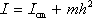This is the parallel axis theorem. Consider an object whose moment of inertia with respect to an axis through its center of mass is known. The moment of inertia, with respect to an axis that is parallel to said axis through the center of mass, is equal to the moment of inertia with respect to the axis through the center of mass, plus the mass of the object times the square of the distance between the two axes.